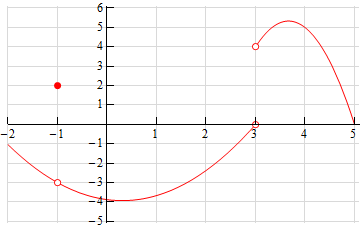Paul's Online Notes
Home / Calculus I / Limits / One-Sided Limits
Show Mobile Notice Show All Notes Hide All Notes
Mobile Notice
You appear to be on a device with a "narrow" screen width (i.e. you are probably on a mobile phone). Due to the nature of the mathematics on this site it is best views in landscape mode. If your device is not in landscape mode many of the equations will run off the side of your device (should be able to scroll to see them) and some of the menu items will be cut off due to the narrow screen width.

### Section 2.3 : One-Sided Limits

4. Sketch a graph of a function that satisfies each of the following conditions.

$\begin{array}{ccl}\mathop {\lim }\limits_{x \to {3^{\, - }}} f\left( x \right) = 0 & \hspace{0.5in}\mathop {\lim }\limits_{x \to {3^{\, + }}} f\left( x \right) = 4 & \hspace{0.5in}f\left( 3 \right){\mbox{ does not exist}}\\ \mathop {\lim }\limits_{x \to - 1} f\left( x \right) = - 3 & \hspace{0.5in} f\left( { - 1} \right) = 2 & \end{array}$ Show Solution

There are literally an infinite number of possible graphs that we could give here for an answer. However, all of them must the following two sets of criteria.

First, at $$x = 3$$ there cannot be a closed dot on the graph (as indicated by the fact that the function does not exist here), the graph must be approaching a value of 0 as it approaches $$x = 3$$ from the left (as indicated by the left-hand limit) and it must be approaching a value of 4 as it approaches $$x = 3$$ from the right (as indicated by the right-hand limit).

Next, the graph must have a closed dot at the point $$\left( { - 1,2} \right)$$ and the graph must be approaching a value of -3 as it approaches $$x = - 1$$ from both sides (as indicated by the fact that value of the overall limit is -3 at this point).

Here is a sketch of one possible graph that meets these conditions.